# Machines

## Exercise 3(A)

#### Question 1

(a) What do you understand by a simple machine?

(b) State the principle of an ideal machine.

(a) A machine is a device by which we can either overcome a large resistive force (or load) at some point by applying a small force (or effort) at a convenient point and in a desired direction or by which we can obtain a gain in speed.

(b) An ideal machine is that in which there is no loss of energy in any manner. Here, the work output is equal to the work input, i.e. its efficiency is 100%.

#### Question 2

State four ways in which machines are useful to us?

Machines are useful to us in —

(a) Changing the point of application of effort to a convenient point.

(b) Lifting a heavy load by applying a less effort.

(c) Changing the direction of effort to a convenient direction.

(d) For obtaining a gain in speed.

#### Question 3

Name a machine for each of the following use —

(a) to multiply the force,

(b) to change the point of application of force,

(c) to change the direction of force,

(d) to obtain the gain in speed.

(a) In order to multiply the force — a bar is used to lift a heavy stone.

(b) In order to change the point of application of force — The rear wheel of a cycle is rotated by applying the effort on the pedal attached to the toothed wheel which is joined to the rear wheel with the help of a chain.

(c) In order to change the direction of force — to lift a bucket full of water from the well, a single fixed pulley is used by applying the effort in the downward direction instead of applying it upwards when the bucket is lifted up without the use of pulley.

(d) In order to obtain gain in speed — A knife. The blade of a knife moves longer by a small displacement of its handle.

#### Question 4

What is the purpose of a jack in lifting a car by it?

A jack is a mechanical lifting device used to lift heavy loads.

A jack works as a force multiplier, as with a little effort on the jack we are able to lift heavy load like a car. It acts as a force multiplier.

#### Question 5

What do you understand by an ideal machine? How does it differ from a practical machine?

An ideal machine is that where there is no loss of energy i.e. the work output is equal to the work input. An ideal machine is 100% efficient.

In a practical machine output energy is always less than the input energy i.e. there is some loss of energy during the operation which is not the case in an ideal machine.

#### Question 6

Explain the term mechanical advantage. State its unit.

The ratio of the load to the effort is called mechanical advantage of the machine.

$\text {Mechanical Advantage} = \dfrac{\text {Load}}{\text {Effort}} \\[0.5em]$

As mechanical advantage is a ratio of similar quantities so it has no unit.

#### Question 7

Define the term velocity ratio. State its unit.

Velocity ratio is defined as the ratio of the velocity of effort to the velocity of the load.

$\text {Velocity Ratio} = \dfrac{\text {Velocity of effort}}{\text {Velocity of load}} \\[0.5em]$

As velocity ratio is a ratio of two similar quantities, so it has no unit.

#### Question 8

How is mechanical advantage related to the velocity ratio for

(i) an ideal machine,

(ii) a practical machine?

(i) For an ideal machine (free from friction etc.), work output is equal to the work input, so the efficiency is equal to 1 ( or 100% ) and the mechanical advantage is numerically equal to the velocity ratio. So,

For an ideal machine, M.A. = V.R.

(ii) In the case of a practical machine, the mechanical advantage is always less than its velocity ratio or the output work is always less than the input work as some of the input energy is lost due to the force of friction etc. So,

For a practical machine, M.A. < V.R.

#### Question 9

Define the term efficiency of a machine. Give two reasons for a machine not to be 100% efficient?

The efficiency of a machine is defined as the ratio of the work done on load by the machine to the work done on the machine by the effort.

$\eta = \dfrac{\text {Work output}}{\text {Work Input}} \\[0.5em]$

It has no unit as it is the ratio of two similar quantities.

A machine is not always 100% efficient due to —

(a) friction

(b) weight of moving parts of machine of a given design.

Due to the above reasons, the velocity ratio of the machine does not change but it's mechanical advantage decreases so its efficiency decreases.

#### Question 10

When does a machine act as

(a) a force multiplier

(b) a speed multiplier?

Can a machine act as a force multiplier and speed multiplier simultaneously?

(a) A machine acts as a force multiplier when the effort arm is longer than the load arm. The mechanical advantage in such cases is greater than 1.

(b) A machine acts as a speed multiplier when the effort arm is shorter than the load arm. The mechanical advantage in such cases is less than 1.

No, it is not possible for a machine to act as a force multiplier and speed multiplier simultaneously.

For a machine used as a force multiplier, effort < load while for a machine used to obtain a gain in speed, effort > load. Hence, it is not possible for a single machine to act as both, simultaneously.

#### Question 11

A machine works as a

(i) force multiplier,

(ii) speed multiplier.

In each case state whether the velocity ratio is more than or less than 1.

(i) When a machine works as a force multiplier then the displacement of the load is less than the displacement of effort. Hence, the velocity ratio is more than 1.

(ii) When a machine works as a speed multiplier then the displacement of load is more than displacement of effort. Hence, the velocity ratio is less than 1.

#### Question 12

(a) State the relationship between mechanical advantage, velocity ratio and efficiency.

(b) Name the term that will not change for a machine of a given design.

(a) The mechanical advantage of a machine is equal to the product of its efficiency and velocity ratio.

M.A. = V.R. x η

(b) The term that does not change for a machine of a given design is its velocity ratio.

#### Question 13

Derive a relationship between mechanical advantage, velocity ratio and efficiency of a machine.

Suppose a machine overcomes a load L by the application of effort E.

Let,

the displacement of effort = dE and

the displacement of the load = dL in time t.

$\text{Work Input} = effort \times \text{displacement of effort} \\[0.5em] = E \times d_E \\[0.5em] \text{Work Output} = load \times \text{displacement of load} \\[0.5em] = L \times d_L \\[0.5em] \text{Efficiency η} = \dfrac{\text{work output}}{\text{work input}} \\[0.5em] η = \dfrac{L \times d_L}{E \times d_E} \\[0.5em] η = \dfrac{L}{E} \times \dfrac{d_L}{d_E} \\[0.5em] = \dfrac{L}{E} \times \dfrac{1}{\dfrac{d_E}{d_L}} \\[0.5em]$

But we know that,

$\dfrac{L}{E} = M.A. \\[0.5em]$

and

$\dfrac{d_E}{d_L} = V.R. \\[0.5em]$

Substituting the above two values in the formula for efficiency we get

$η = \dfrac{M.A.}{V.R.} \\[0.5em] \Rightarrow M.A. = V.R. \times η \\[0.5em]$

Hence, the mechanical advantage of a machine is equal to the product of its efficiency and velocity ratio.

#### Question 14

How is mechanical advantage related to the velocity ratio for an actual machine? State whether the efficiency of such a machine is equal to 1, less than 1 or more than 1.

For an actual machine, the mechanical advantage is always less than its velocity ratio or the output work is always less than the input work.

The efficiency of such a machine is always less than 1 as some part of input energy is lost against the force of friction.

#### Question 15

State one reason why mechanical advantage is less than the velocity ratio for an actual machine.

The mechanical advantage of an actual machine is always less than the velocity ratio as there is a loss of energy due to friction.

#### Question 16

What is a lever? State its principle.

A lever is a rigid, straight (or bent) bar which is capable of turning about a fixed axis.

A lever works on the principle of moments, according to which in the equilibrium position of levers, moment of load about the fulcrum must be equal to the moment of effort about the fulcrum and the two moments must be in opposite directions.

#### Question 17

Write down a relation expressing the mechanical advantage of a lever.

The expression of the mechanical advantage of a lever is:

$M.A. = \dfrac{\text{Effort arm}}{\text{Load arm}} \\[0.5em]$

#### Question 18

Name the three classes of levers and state how are they distinguished. Give two examples of each class.

The three classes of levers are —

(i) Class I levers — In this type of the levers, the fulcrum F is in between the effort E and the load L.

Example — a seesaw, a pair of scissors

(ii) Class II levers — In these type of levers, the fulcrum F and the effort E are at the two ends of the lever and the load L is somewhere in between the effort E and the fulcrum F.

Example — a nutcracker, a bottle opener.

(iii) Class III levers — In these type of levers, the fulcrum F and the load L are at the two ends of the lever and the effort E is somewhere in between the fulcrum F and the load L.

Example — sugar tongs, foot treadle.

#### Question 19

Give one example each of a class I lever where the mechanical advantage is

(a) more than 1, and

(b) less than 1.

(a) For a class I lever, the mechanical advantage is more than 1 in the case of shears used for cutting the thin metal sheets.

(b) For a class I lever, the mechanical advantage is less than 1 in case of a pair of scissors whose blades are longer than its handles.

#### Question 20

What is the use of a lever if its mechanical advantage is

(a) more than 1,

(b) equal to 1, and

(c) less than 1?

(a) When the mechanical advantage is more the 1, the lever serves as force multiplier i.e. it enables us to overcome a large resistive force (load) by a small effort.

(b) When the mechanical advantage is equal to 1, the lever has effort arm and load arm of equal lengths like in a physical balance with both arms equal in length.

(c) When the mechanical advantage is less than 1, the levers are used to obtain the gain in speed. This means that the displacement of load is more as compared to the displacement of effort.

#### Question 21

Both a pair of scissors and a pair of pliers belong to the same class of levers. Name the class of lever. Which one has the mechanical advantage less than 1?

A pair of scissors and a pair of pliers both belong to class I lever.

As the blades of the scissors are longer than its handles, so the effort arm is shorter than the load arm. Hence, the mechanical advantage of a pair of scissors is less than 1.

#### Question 22

Explain why scissors for cutting cloth may have blades longer than the handles, but shears for cutting metals have short blades and long handles.

A pair of scissors which is used to cut a piece of cloth has blades longer than the handles i.e. it has effort arm shorter than load arm so that the blades move longer on the cloth when the handles are moved a little.

Shears used for cutting metals have short blades and long handles i.e. it's effort arm is longer than the load arm and acts as a force multiplier which helps us to overcome large resistive force by a small effort.

#### Question 23

Figure shows a uniform metre rule of weight W supported on a fulcrum at the 60 cm mark by applying the effort E at the 90 cm mark.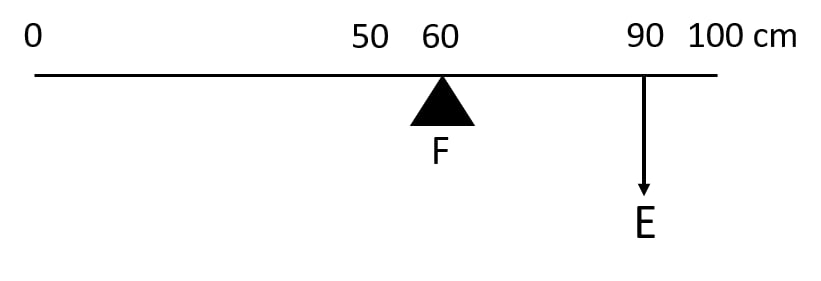(a) State with reasons whether the weight W of the rule is greater than, less than or equal to the effort E.

(b) Find the mechanical advantage in an ideal case.

(a) The weight W of the scale is greater than E.

The effort arm is 30 cm and the load arm is 10 cm. In order to balance the scale — weight (W) of scale has to be more than effort (E).

(b) Given,

Effort arm = 30 cm

As we know that,

$M.A. = \dfrac{\text{Effort arm}}{\text{Load arm}} \\[0.5em]$

Substituting the values in the formula we get,

$M.A. = \dfrac{\text{30}}{\text{10}} \\[0.5em] \Rightarrow M.A. = 3$

#### Question 24

Which type of lever has a mechanical advantage always more than 1? Give reason with one example. What change can be made in this lever to increase its mechanical advantage?

The Class II levers have mechanical advantage always greater than 1 and the reason for this is that, the load and effort are on same side of the fulcrum but in opposite directions and the effort arm is always greater than the load arm.

Therefore, mechanical advantage is always greater than 1.

This type of levers act as a force multiplier so less effort is needed to overcome a large load.

Example — a nut cracker

In order to increase the mechanical advantage, we can increase the length of effort arm.

#### Question 25

Draw a diagram of a lever which is always used as a force multiplier. How is the effort arm related to the load arm in such a lever?

A Class II lever acts as a force multiplier as the mechanical advantage is always greater than 1 and the effort arm is longer than the load arm.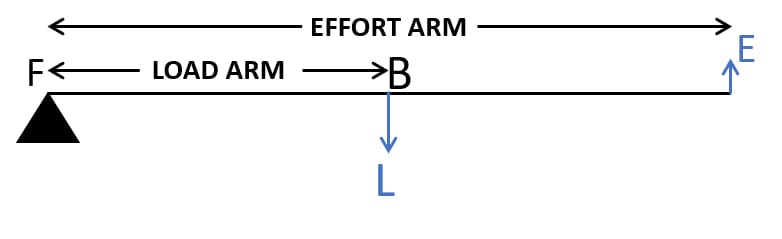#### Question 26

Explain why the mechanical advantage of a class II type of lever is always more than 1.

In Class II levers, the fulcrum F and the effort E are at the two ends of the lever and the load L is somewhere in between the effort E and the fulcrum F.

So, the load and the effort are on the same side of the fulcrum but in opposite directions and the effort arm is always longer than the load arm.

Therefore, the mechanical advantage is always greater than 1.

#### Questions 27

Draw a labelled diagram of a Class II lever. Give one example of such a lever.

The figure below represents a Class II lever.A nut cracker is an example of a class II lever.

#### Question 28

Figure shows a lemon crusher.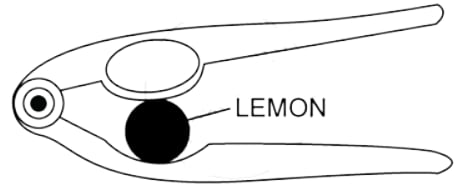(a) In the diagram, mark the position of the directions of load L and effort E.

(b) Name the class of lever.

(a) Labelled diagram of lemon crusher is shown below: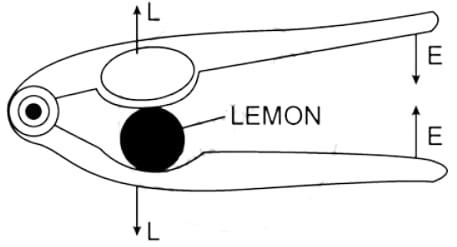(b) It is a class II lever.

#### Question 29

The diagram below shows a rod lifting a stone.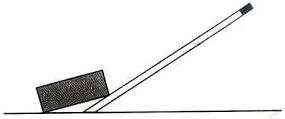(a) Mark position of fulcrum F and draw arrows to show the directions of load L and effort E.

(b) What class of lever is the rod?

(c) Give one more example of the same class of lever stated in part (b).

(a) Below diagram shows the position of fulcrum F and the directions of load L and effort E: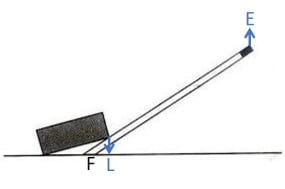(b) The rod is a Class II lever as the load is between fulcrum and effort.

(c) A nut cracker is also an example of a Class II lever.

#### Question 30

State the kind of lever which always has the mechanical advantage less than 1. Draw a labelled diagram of such a lever.

The Class III levers have mechanical advantage always less than 1.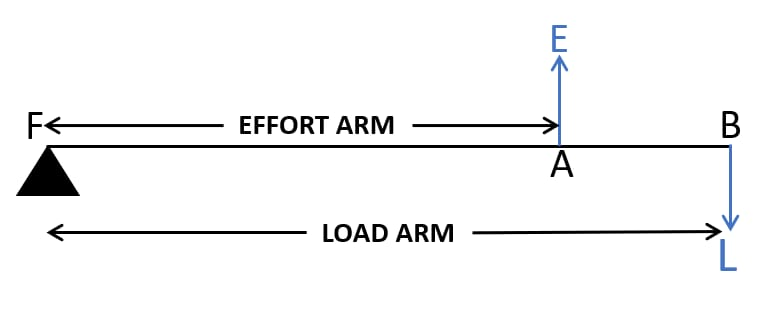#### Question 31

Explain why the mechanical advantage of the class III lever is always less than 1.

The fulcrum F and the load L are at the two ends of the lever and the effort E is somewhere in between the fulcrum F and the load L.

The effort and the load are on the same side of fulcrum but in opposite directions and the effort arm is always smaller than the load arm. Therefore, the mechanical advantage of class III lever is always less than 1.

#### Question 32

Classes III levers have mechanical advantage less than 1. Why are they then used?

Class III levers have mechanical advantage less than 1 so as effort arm is always less than the load arm, so we do not get gain in force, but we get gain in speed, i.e. a larger displacement of load is obtained by a smaller displacement of effort.

#### Question 33

Draw a labelled sketch of a class III lever. Give one example of this kind of lever.Sugar tongs is an example of class III lever.

#### Question 34

State the class of levers and the relative positions of load (L), effort (E) and fulcrum (F) in

(a) a bottle opener, and

(b) sugar tongs.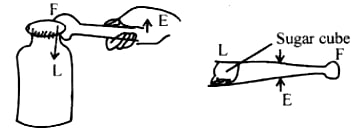(a) A bottle opener is an example of Class II lever, as the fulcrum F and the effort E are at the two ends of the lever and the load is in between the fulcrum F and the effort E.

(b) Sugar tongs is an example of Class III lever, as the fulcrum F and the load L are at the two ends of the lever and the effort E is in between the fulcrum F and the load L.

#### Question 35

Draw diagrams to illustrate the position of fulcrum, load and effort, in each of the following:

(a) A seesaw

(b) A common balance

(c) A nutcracker

(d) Forceps

(a) A seesaw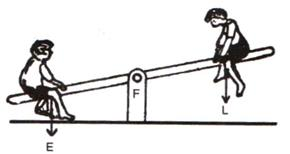(b) A common balance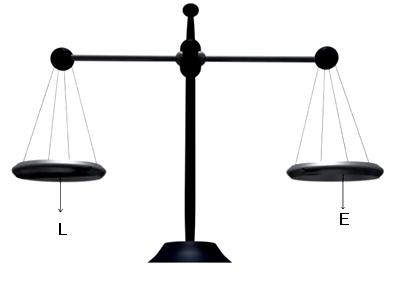(c) A nutcracker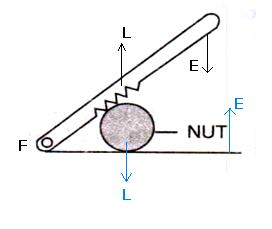(d) Forceps.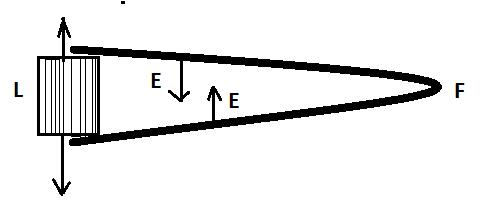#### Question 36

Classify the following into levers as class I, class II or class III:

(a) A door

(b) A catapult

(c) Claw hammer

(d) A wheel barrow

(e) A fishing rod

(f) Sugar tongs

(a) A door — Class II

(b) A catapult — Class I

(c) Claw hammer — Class I

(d) A wheel barrow — Class II

(e) A fishing rod — Class III

(f) sugar tongs — Class III

#### Question 37

What type of lever is formed by the human body while

(a) raising a load on the palm, and

(b) raising the weight of body on toes?

(a) When a human body raises a load on the palm then it is an example of a Class III lever. The elbow joint acts as fulcrum F at one end, biceps exerts the effort in the middle and load L on the palm is at the other end.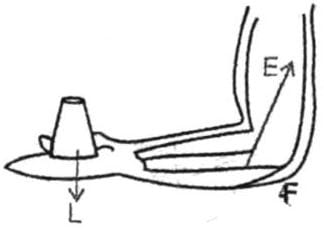(b) When raising the weight of body on toes then it is a Class II lever. The fulcrum F is at toes at one end, the load L (i.e. weight of the body) is in the middle and the effort E by muscles is at the other end.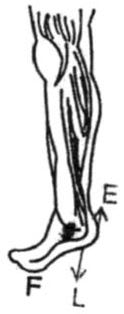#### Question 38

Indicate the positions of load L, effort E and fulcrum F in the forearm shown below. Name the class of lever.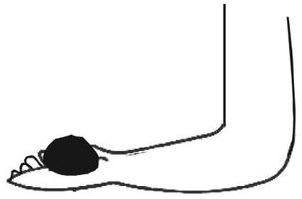The positions of load L, effort E and fulcrum F in the forearm are shown below: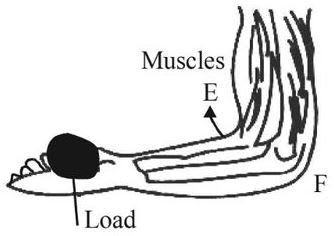It is a Class III lever.

#### Question 39

Give an example of each class of lever in a human body.

The examples of each class of lever in a human body are:

(i) The action of nodding of the head is a Class I lever. In this case the spine acts as the fulcrum F, load L is at its front part while effort E is at its rear part.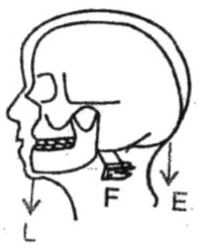(ii) The action of raising the weight of the body on toes is an example of Class II lever. In this case, the fulcrum F is at toes at one end, the load L (i.e. the weight of the body) is in the middle and effort E by muscles is at the other end.(iii) The action of raising a load by forearm is an example of Class III lever. In this case the elbow joint acts as fulcrum F at one end, biceps exerts the effort E in the middle and a load L on the palm is at the other end.#### Question 40

Complete the following sentences:

(a) Mechanical advantage = ...... × velocity ratio

(b) In class II lever, effort arm is ...... than the load arm.

(c) A pair of scissors is a ...... multiplier.

(a) Mechanical advantage = efficiency × velocity ratio

(b) In Class II lever, effort arm is longer than the load arm.

(c) A pair of scissors is speed multiplier

## Multiple Choice Type

#### Question 1

1. M.A. = L x E
2. M.A. x E = L ✓
3. E = M.A. x L
4. None of these

#### Question 2

The correct relationship between the mechanical advantage (M.A.), velocity ratio (V.R.) and efficiency (η) is:

1. M.A. = η x V.R. ✓
2. V.R. = η x M.A.
3. η = M.A. x V.R.
4. None of these

#### Question 3

Select the incorrect statement:

1. A machine always has efficiency less than 100%.
2. The mechanical advantage of a machine can be less than 1.
3. A machine can be used as a speed multiplier.
4. A machine can have mechanical advantage greater than the velocity ratio. ✓

#### Question 4

The lever for which mechanical advantage is less than 1 has:

1. fulcrum at the mid-point between load and effort.
2. load between effort and fulcrum.
3. effort between fulcrum and load. ✓
4. load and effort acting at the same point.

#### Question 5

Class II levers are designed to have:

1. M.A. = V.R.
2. M.A. > V.R.
3. M.A. > 1 ✓
4. M.A. < 1

## Numericals

#### Question 1

A crowbar of length 120 cm has its fulcrum situated at a distance of 20 cm from the load. Calculate the mechanical advantage of the crowbar.

Given,

Total length of a crowbar = 120 cm

Effort arm = 120 – 20 = 100 cm

We know that,

$\text{Mechanical advantage M.A.} = \dfrac{\text{Effort arm}}{\text{Load arm}} \\[0.5em] M.A. = \dfrac{100}{20} \\[0.5em] \Rightarrow M.A. = 5 \\[0.5em]$

#### Question 2

A pair of scissors has its blades 15 cm long, while its handles are 7.5 cm long. What is its mechanical advantage?

Given,

Effort arm = 7.5 cm

$\text{Mechanical advantage M.A.} = \dfrac{\text{Effort arm}}{\text{Load arm}} \\[0.5em] M.A. = \dfrac{7.5}{15} \\[0.5em] \Rightarrow M.A. = 0.5 \\[0.5em]$

#### Question 3

A force of 5kgf is required to cut a metal sheet. A pair of shears used for cutting the metal sheet has its blades 5 cm long, while its handles are 10 cm long. What effort is needed to cut the sheet?

Given,

Effort arm = 10 cm

$\text{Mechanical advantage M.A.} = \dfrac{\text{Effort arm}}{\text{Load arm}} \\[0.5em] M.A. = \dfrac{10}{5} \\[0.5em] \Rightarrow M.A. = 2 \\[0.5em]$

We also know that,

$\text{Effort} = \dfrac{Load}{M.A.} \\[0.5em]$

Substituting the values in the formula we get,

$\text{Effort} = \dfrac{5}{2} \\[0.5em] \Rightarrow \text{Effort} = \text {2.5 kgf}$

#### Question 4

The diagram below shows a lever in use.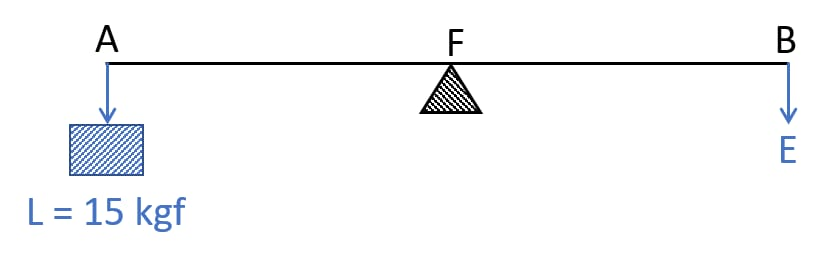(a) To which class of lever does it belong?

(b) If AB =1 m, AF= 0.4 m, find its mechanical advantage.

(c) Calculate the value of E.

(a) The diagram represents a Class I lever.

(b) Given,

AB = 1m

AF = Load arm =0.4 m

BF = Effort arm = 0.6 m

$\text{Mechanical advantage M.A.} = \dfrac{\text{Effort arm}}{\text{Load arm}} \\[0.5em] M.A. = \dfrac{0.6}{0.4} \\[0.5em] \Rightarrow M.A. = 1.5 \\[0.5em]$

(c) We know that,

$\text{Effort} = \dfrac{Load}{M.A} \\[0.5em]$

Given,

Substituting the values in the formula we get,

$\text{Effort} = \dfrac{15}{1.5} \\[0.5em] \Rightarrow \text{Effort} = \text{10kgf} \\[0.5em]$

#### Question 5

A man uses a crowbar of length 1.5 m to raise a load of 75 kgf by putting a sharp edge below the bar at a distance 1 m from his hand.

(a) Draw a diagram of the arrangement showing the fulcrum (F), load (L) and effort (E) with their directions.

(b) State the kind of lever.

(c) Calculate — (i) load arm, (ii) effort arm, (iii) mechanical advantage, and (iv) the effort needed.

(a) Below is the diagram of the crowbar showing the fulcrum (F), load (L) and effort (E) with their directions: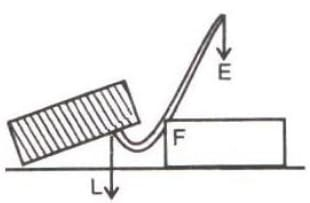(b) Crowbar belongs to class I lever.

Given,

(i) Total length = 1.5 m

Effort arm = 1 m

$\text{Load arm} = \text{Total length} - \text{Effort arm} \\[0.5em] \text{Load arm} = 1.5m - 1m \\[0.5em] \Rightarrow \text{Load arm} = 0.5 m \\[0.5em]$

(ii) Effort arm = 1 m

(iii)

$\text{Mechanical advantage M.A.} = \dfrac{\text{Effort arm}}{\text{Load arm}} \\[0.5em] M.A. = \dfrac{1}{0.5} \\[0.5em] \Rightarrow M.A. = 2 \\[0.5em]$

(iv) Given,

We know that,

$\text{Effort} =\dfrac{\text{Load}}{\text{M.A.}} \\[0.5em]$

Substituting the values in formula we get,

$\text{Effort} =\dfrac{75}{2} \\[0.5em] \Rightarrow \text{Effort} = 37.5 \text{ kgf} \\[0.5em]$

#### Question 6

A pair of scissors is used to cut a piece of cloth by keeping it at a distance of 8.0 cm from its rivet and applying an effort of 10 kgf by fingers at a distance 2.0 cm from the rivet.

(a) Find: (i) the mechanical advantage of scissors and (ii) the load offered by the cloth.

(b) How does the pair of scissors act - as a force multiplier or as a speed multiplier?

(a)

(i) We know that,

$\text{Mechanical advantage M.A.} = \dfrac{\text{Effort arm}}{\text{Load arm}} \\[0.5em]$

Given,

Effort arm = 2 cm

Substituting the values in the formula we get,

$M.A. = \dfrac{2}{8} \\[0.5em] \Rightarrow M.A. = 0.25 \\[0.5em]$

(ii) We know,

$Load = M.A. \times Effort \\[0.5em]$

Given,

Effort = 10 kgf

Substituting the values in the formula we get,

$Load = 0.25 \times 10 \\[0.5em] \Rightarrow Load = 2.5 kgf \\[0.5em]$

(b) As the mechanical advantage is less than 1 i.e. M.A. < 1. Hence, the pair of scissors acts as a speed multiplier as there is larger displacement of load than displacement of effort.

#### Question 7

A 4 m long rod of negligible weight is supported at a point 125 cm from its one end and a load of 18 kgf is suspended at a point 60 cm from the support on the shorter arm.

(a) If a weight W is placed at a distance of 250 cm from the support on the longer arm to balance the rod, find W.

(b) If a weight 5 kgf is kept to balance the rod, find its position.

(c) To which class of lever does it belong?

(a) The principle of moments states that —

Given,

Load = 18kgf and 18 kgf load is placed at 60 cm from the support.

Effort = W and W kgf weight is placed at 250 cm from the support.

Substituting the values in the formula we get,

$18 \times 60 = W \times 250 \\[0.5em] \Rightarrow W = \dfrac{18 \times 60}{250} \\[0.5em] \Rightarrow W = 4.32 \\[0.5em]$

∴ W = 4.32 kgf

(b) The principle of moments states that —

Given,

Load = 18kgf and 18 kgf load is placed at 60 cm from the support.

W = 5 kgf and 5 kgf weight is placed at d cm from the support.

Substituting the values in the formula we get,

$18 \times 60 = 5\times d \\[0.5em] \Rightarrow d = \dfrac{18 \times 60}{5} \\[0.5em] \Rightarrow d = 216 cm \\[0.5em]$

∴ d = 216 cm

(c) It belongs to class I lever as fulcrum is in between the effort and the load.

#### Question 8

A lever of length 9 cm has its load arm 5 cm long and the effort arm is 9 cm long.

(a) To which class does it belong?

(b) Draw diagram of the lever showing the position of fulcrum F and directions of both the load L and effort E.

(c) What is the mechanical advantage and velocity ratio if the efficiency is 100%?

(d) What will be the mechanical advantage and velocity ratio if the efficiency becomes 50%?

(a) It belongs to Class II lever as length of the lever is equal to the effort arm and the effort arm is also more than the load arm.

(b) Below is the diagram of the lever with position of fulcrum F and directions of both the load L and effort E labelled: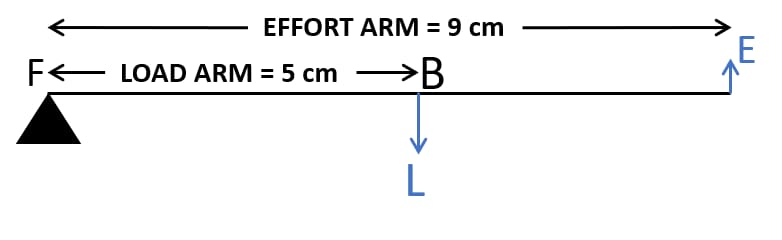(c) We know that,

$\text{Mechanical advantage M.A.} = \dfrac{\text{Effort arm}}{\text{Load arm}} \\[0.5em]$

Given,

Effort arm = 9 cm

Substituting the values in the formula we get,

$M.A. = \dfrac{9}{5} \\[0.5em] \Rightarrow M.A. = 1.8 \\[0.5em]$

Relation between mechanical advantage, efficiency and velocity ratio is

$M.A. = \eta \times V.R. \\[0.5em]$

Given, η = 100% = 1

So,

$M.A. = 1 \times V.R. \\[0.5em]$

Therefore, Mechanical advantage = Velocity ratio = 1.8

(d) When efficiency reduces to 50%, its mechanical advantage reduces however, its velocity ratio remains the same. So,

M.A. = 0.5 x 1.8 = 0.9
V.R. = 1.8

#### Question 9

The diagram below shows a lever in use.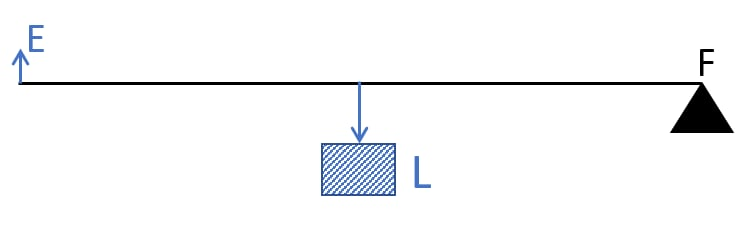(a) To which class of lever does it belong?

(b) Without changing the dimensions of the lever, if the load is shifted towards the fulcrum what happens to the mechanical advantage of the lever?

(a) The diagram shown belongs to class II lever as Load is in between Fulcrum and Effort.

(b) The mechanical advantage of the lever increases when load is shifted towards the fulcrum, as less effort is required to lift the load.

#### Question 10

The figure below shows a wheel barrow of mass 15 kg carrying a load of 30 kgf with its centre of gravity at A. The points B and C are the centre of wheel and tip of the handle such that the horizontal distance AB = 20 cm and AC = 40 cm.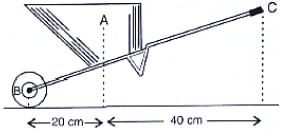Find:

(b) the effort arm,

(d) the minimum effort required to keep the leg just off the ground.

(a) Load arm AF = 20 cm

(b) Effort arm CF = 60 cm

(c) We know that,

$\text{Mechanical advantage M.A.} = \dfrac{\text{Effort arm}}{\text{Load arm}} \\[0.5em]$

Substituting the values in the formula we get,

$M.A. = \dfrac{60}{20} \\[0.5em] \Rightarrow M.A. = 3 \\[0.5em]$

(d) We know that,

$\text{Effort} = \dfrac{\text{Load}}{\text{M.A.}} \\[0.5em]$

Given,

Load = 30 kgf + 15 kgf = 45 kgf

Substituting the values in the formula we get,

$\text{Effort} = \dfrac{45}{3} \\[0.5em] \Rightarrow \text{Effort} = 15\text{ kgf} \\[0.5em]$

#### Question 11

The diagram below shows the use of a lever.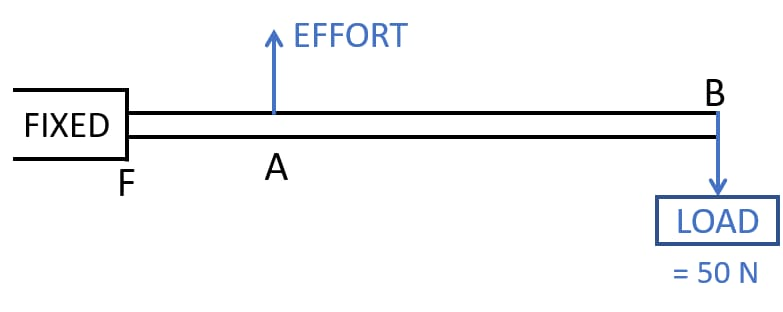(a) State the principle of moments as applied to the above lever.

(b) To which class of lever does it belong? Give an example of this class of lever.

(c) If FA = 10 cm, AB = 490 cm,

calculate:

(ii) the minimum effort required to lift the load (= 50N).

(a) The principle of moments states that —

(b) The above diagram belongs to Class III lever. The example of Class III lever is a sugar tongs.

(c)

(i) We know that,

$\text{Mechanical advantage M.A.} = \dfrac{\text{Effort arm}}{\text{Load arm}} \\[0.5em]$

Given,

Load arm = 490 cm + 10 cm = 500 cm

Effort arm = 10 cm

Substituting the values in the formula we get,

$M.A. = \dfrac{10}{500} \\[0.5em] \Rightarrow M.A. = \dfrac{1}{50} \\[0.5em]$

(ii) We know that,

$\text{Effort} = \dfrac{\text{Load}}{\text{M.A.}} \\[0.5em]$

Given,

Substituting the values in the formula we get,

$\text{Effort} = \dfrac{50}{\dfrac{1}{50}} \\[0.5em] \Rightarrow \text{Effort} = 2500 N \\[0.5em]$

#### Question 12

A fire tongs has its arms 20 cm long. It is used to lift a coal of weight 1.5 kgf by applying an effort at a distance 15 cm from the fulcrum.

Find:

(i) the mechanical advantage of fire tongs and

(ii) the effort needed.

We know that,

$\text{Mechanical advantage M.A.} = \dfrac{\text{Effort arm}}{\text{Load arm}} \\[0.5em]$

Given,

Effort arm = 15 cm

Substituting the values in the formula we get,

$M.A. = \dfrac{15}{20} \\[0.5em] \Rightarrow M.A. = 0.75 \\[0.5em]$

(ii) We know that,

$\text{Effort} = \dfrac{\text{Load}}{\text{M.A.}} \\[0.5em]$

Given,

Substituting the values in the formula we get,

$\text{Effort} = \dfrac{1.5}{0.75} \\[0.5em] \Rightarrow \text{Effort} = 2 \text{ kgf} \\[0.5em]$

## Exercise 3(B)

#### Question 1

What is a fixed pulley? State its one use.

A pulley, which has its axis of rotation stationary in position is called a fixed pulley.

A fixed pulley is used to change the direction of effort to be applied. It is used for lifting small load such as a water bucket.

#### Question 2

What is the ideal mechanical advantage of a single fixed pulley? Can it be used as a force multiplier?

In case of an ideal single fixed pulley the effort needed is equal to the load itself and so the mechanical advantage is equal to 1.

As the amount of effort required is equal to the load itself so a single fixed pulley cannot be used as a force multiplier.

#### Question 3

Name the pulley which has no gain in mechanical advantage. Explain, why is such a pulley then used?

The pulley that has no gain in mechanical advantage is a single fixed pulley.

A single fixed pulley is used only to change the direction of effort to be applied i.e. with its use the effort can be applied in a more convenient direction.

It is difficult to apply the effort upwards to lift a load up directly, but it becomes easier with the help of a fixed pulley, because the effort can be applied in the downward direction to raise the load up. Further to apply the effort downwards one can conveniently make use of his own weight also for the effort.

#### Question 4

What is the velocity ratio of a single fixed pulley?

The velocity ratio of a single fixed pulley is 1. If the point of application of effort E moves a distance d, then the load L also moves the same distance d upwards.

i.e. if dE = dL = d then V.R. = 1

$V.R. = \dfrac{d_E}{d_L} = \dfrac{d}{d} = 1 \\[0.5em]$

#### Question 5

In a single fixed pulley, if the effort moves by a distance x downwards, by what height is the load raised upwards?

If the point of application of effort E moves a distance x downwards, then the load L also moves the same distance x upwards.

#### Question 6

What is a single movable pulley? What is its mechanical advantage in the ideal case?

A pulley whose axis of rotation is movable is called a single movable pulley. The mechanical advantage in the ideal case is 2 as the load can be lifted by applying the effort equal to half the load. A single, movable pulley acts as a force multiplier.

#### Question 7

Name the type of a single pulley that has an ideal mechanical advantage equal to 2. Draw a labelled diagram of the pulley mentioned by you.

The single movable pulley acts as a force multiplier and has a mechanical advantage equal to 2. Below is the labelled diagram of a single movable pulley: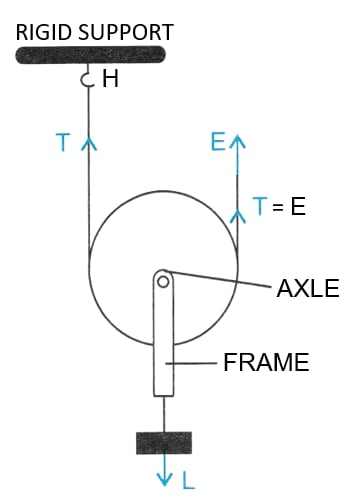#### Question 8

Give two reasons why the efficiency of a single movable pulley is not 100%.

The efficiency of a single movable pulley system is not 100% because of the following reasons:

1. There is friction in the pulley bearings or at the axle.
2. The weight of the pulley and string is not zero.

#### Question 9

In which direction does the force need to be applied, when a single pulley is used with a mechanical advantage greater than 1? How can you change the direction of force applied without altering its mechanical advantage? Draw a labelled diagram of the system.

In the case of a single pulley with a mechanical advantage greater than 1, the force needs to be applied in an upward direction which is very inconvenient.

In order to change the direction of force applied without altering its mechanical advantage, we have to use a single movable pulley along with a fixed pulley.

The load is attached to the axle of movable pulley A and the effort is applied in the downward direction at the free end of the string passing over the fixed pulley B. One can also use his own weight as effort which will be quite convenient.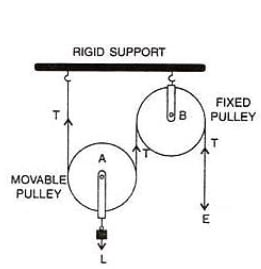#### Question 10

What is the velocity ratio of a single movable pulley? How does the friction in the pulley bearing affect it?

In the case of a single movable pulley the velocity ratio is always 2. The friction in the pulley bearing has no effect.

#### Question 11

In a single movable pulley, if the effort moves by a distance x upwards, by what height is the load raised?

In a single movable pulley, if the effort moves by a distance x upward, the load is raised to a height of $\dfrac{x}{2}$ as the segment of string on both sides of the pulley moves up by a distance $\dfrac{x}{2}$.

#### Question 12

Draw a labelled diagram of an arrangement of two pulleys, one fixed and other moveable. In the diagram, mark the directions of all forces acting on it. What is the ideal mechanical advantage of the system? How can it be achieved?

Below is the diagram of an arrangement of two pulleys, one fixed and other moveable:The ideal mechanical advantage of a single movable pulley with a fixed pulley system is 2. The ideal mechanical advantage of 2 can be achieved by assuming there is no friction in the pulley bearings, between the string and surface of the rim of the pulley and that string and the pulley are massless.

#### Question 13

The diagram alongside shows a pulley arrangement.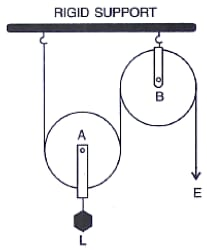(a) Name the pulleys A and B.

(b) In the diagram, mark the direction of tension on each strand of string.

(c) What is the purpose of the pulley B?

(d) If the tension is T, deduce the relation between (i) T and E, and (ii) E and L.

(e) What is the velocity ratio of the arrangement?

(f) Assuming that the efficiency of the system is 100%, what is the mechanical advantage?

(a) A → Movable pulley, B → Fixed pulley

(b) Tension on each strand of string is as shown in the diagram below:(c) The purpose of fixed pulley B is to change the direction of effort to be applied from upward to downward.

(d)(i) When tension in the string is T then the effort E balances the tension T. So, E = T.

(d)(ii) When the load is balanced by the tension T in two segments of the string and the effort E balances the tension T at the free end, so

L = T + T = 2 T and

E = T,

Hence, 2E = L.

(e) When distance moved by effort is d then distance moved by load is d/2 so the velocity ratio becomes 2.

(f) If the efficiency of the system is 100%, then effort applied is equal to half the load. Hence, it acts as a force multiplier and the mechanical advantage is 2.

#### Question 14

State four differences between a single fixed pulley and a single movable pulley.

Single fixed pulleySingle movable pulley
It is fixed to a rigid support.It is not fixed to a rigid support.
Its velocity ratio is 1.Its velocity ratio is 2.
The weight of pulley itself does not affect its mechanical advantage.The weight of pulley itself reduces its mechanical advantage.
It is used to change the direction of effort from upwards to downwards.It is used as a force multiplier.

#### Question 15

The diagram alongside shows an arrangement of three pulleys A, B and C. The load is marked as L and the effort as E.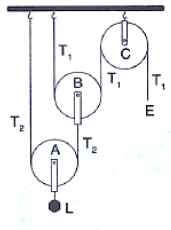(a) Name the pulleys A, B and C.

(b) Mark in the diagram the direction of load (L), effort (E) and tension T1 and T2 in the two strings.

(c) How are the magnitudes of L and E related to the tension T1?

(d) Calculate the mechanical advantage and velocity ratio of the arrangement.

(e) What assumptions have you made in parts (c) and (d)?

(a) Pulleys A and B are movable pulleys while pulley C is a fixed pulley.

(b) The direction of load (L), effort (E) and tension T1 and T2 in the two strings are marked in the below diagram: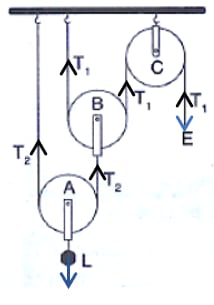(c) The relation between magnitudes of L and E to the tension T1 is as follows:

The magnitude of effort E = T1
The magnitude of load L = 22 T1 = 4T1.

(d) The mechanical advantage is 22 = 4 as there are two movable pulleys and we know,

M.A. = 2n where, n is the number of movable pulleys.

The velocity ratio = 22 = 4 as there are two movable pulleys and we know,

V.R. = 2n where, n is the number of movable pulleys.

1. The pulleys A and B are weightless
2. There is no friction between the bearings of the pulleys or the axle.

#### Question 16

Draw a diagram of combination of three movable pulleys and one fixed pulley to lift up a load. In the diagram, show the directions of load, effort and tension in each strand. Find:

(ii) velocity ratio and

(iii) the efficiency of the combination in the ideal situation.

The diagram is shown below: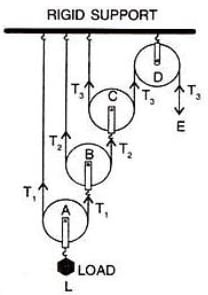In equilibrium,

Effort E = T3       (1)

Tension T1 in the string passing over the pulley A is given as 2T1 = L

T1 = $\bold{\dfrac{L}{2}}$       (2)

Tension T2 in the string passing over the pulley B is given as

2T2 = T1

T2= $\dfrac{T_1}{2}$

Substituting value of T1 from equation 2,

T2 = $\bold{\dfrac{L}{2^2}}$       (3)

Tension T3 in the string passing over the pulley C is given as

2T3 = T2

T3 = $\dfrac{T_2}{2}$

Substituting value of T2 from equation 3,

T3 = $\bold{\dfrac{L}{2^3}}$       (4)

In equilibrium, T3 = E

From equation 4,

Load L = 23 x T3       (5)

$\text{Mechanical advantage M.A.} = \dfrac{L}{E} \\[0.5em] M.A. = \dfrac{2^3 \times T_3}{T^3} \\[0.5em] \Rightarrow M.A. = 2^3$

(ii) As we know, one end of each string passing over a movable pulley is fixed, so the other end of string moves up twice the distance moved by the axle of the movable pulley.

If the load L attached to the pulley A moves a distance d,

then dL = d

Now, the string connected to the axle of pulley B, moves up by a distance,

2 times d = 2d.

Then the string connected to the axle of the pulley C, moves up by a distance,

2 times 2d = 22d

Then the end of the string passing over the fixed pulley D, moves up by a distance,

2 times 22d = 23d.

Hence, dE = 23d.

As we know,

$\text{Velocity Ratio V.R.} = \dfrac{\text{Distance moved by effort }}{\text{Distance moved by load}} \\[0.5em] = \dfrac{d_E}{d_L} \\[0.5em]$

Substituting the values in the formula we get,

$= \dfrac {2^3 \times d}{d} \\[0.5em] = 2^3 \\[1.5em] \text{Efficiency} (\eta) = \dfrac{M.A.}{V.R.} \\[0.5em] = \dfrac{2^3}{2^3} = 1 \\[0.5em]$

#### Question 17

Draw a diagram of a block and tackle system of pulleys having a velocity ratio of 5. In your diagram indicate clearly the points of application and the directions of the load L and effort E. Also mark the tension T in each strand.

The diagram of a block and tackle system of pulleys having a velocity ratio of 5 with directions of the load L, effort E and tension T in each strand is shown below: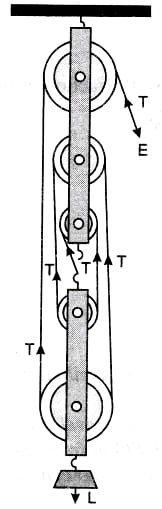#### Question 18

Give reasons for the following —

(a) In a single fixed pulley, the velocity ratio is always more than the mechanical advantage.

(b) The efficiency of a movable pulley is always less than 100%.

(c) In case of a block and tackle system, the mechanical advantage increases with the increase in the number of pulleys.

(d) The lower block of a block and tackle pulley system must be of negligible weight.

(a) In case of a single fixed pulley, the velocity ratio is always more than the mechanical advantage as a little effort is wasted in overcoming the friction between the strings and the grooves of the pulley. Hence, the required effort is greater than the load. As a result velocity ratio is always more than the mechanical advantage.

(b) As some effort is wasted in overcoming the friction between the strings and the grooves of the pulley as a result the efficiency of a movable pulley is always less than 100%.

(c) In the case of a block and tackle system, the mechanical advantage increases with the increase in the number of pulleys.

$M.A. = \dfrac{Load}{Effort} \\[0.5em] M.A. = \dfrac{nT}{T} = n \\[0.5em]$

∴ M.A. = Total number of pulleys in both the blocks

(d) The lower block of a block and tackle pulley system must be of negligible weight as efficiency is reduced due to the weight of the lower block of the pulley.

$\eta = 1 - \dfrac{w}{nE} \\[0.5em]$

where, w is the total weight of the lower block along with the pulleys in it.

#### Question 19

Name a machine which is used to:

(a) multiply force

(b) multiply speed, and

(c) change the direction of force applied.

(a) Multiply force — a movable pulley is used

(b) Multiply speed — Class III lever

(c) Change the direction of force applied — single fixed pulley

#### Question 20

State whether the following statements are true or false.

(a) The velocity ratio of a single fixed pulley is always more than 1.

(b) The velocity ratio of a single movable pulley is always 2.

(c) The velocity ratio of a combination of n movable pulleys with a fixed pulley is always 2n.

(d) The velocity ratio of a block and tackle system is always equal to the number of strands of the tackle supporting the load.

(a) The velocity ratio of a single fixed pulley is always more than 1
False

(b) The velocity ratio of a single movable pulley is always 2
True

(c) The velocity ratio of a combination of n movable pulleys with a fixed pulley is always 2ⁿ
True

(d) The velocity ratio of a block and tackle system is always equal to the number of strands of the tackle supporting the load
True

## Multiple Choice Type

#### Question 1

A single fixed pulley is used because it:

1. has a mechanical advantage greater than 1
2. has a velocity ratio less than 1
3. gives 100% efficiency
4. helps to apply the effort in a convenient direction. ✓

#### Question 2

The mechanical advantage of an ideal single movable pulley is:

1. 1
2. 2 ✓
3. less than 2
4. less than 1.

#### Question 3

A movable pulley is used as :

1. a force multiplier ✓
2. a speed multiplier
3. a device to change the direction of effort
4. an energy multiplier

## Numericals

#### Question 1

A woman draws water from a well using a fixed pulley. The mass of the bucket and water together is 6 kg. The force applied by the women is 70 N. Calculate the mechanical advantage. (Take g = 10 m s-2).

Given,

Load = m x g = 6 x 10 = 60 N

Effort = 70 N

As we know that,

$M.A. = \dfrac{\text{Load}}{\text{Effort}} \\[0.5em]$

Substituting the values in the formula we get,

$M.A. = \dfrac{60}{70} \\[0.5em] M.A. = \dfrac{6}{7} \\[0.5em] \Rightarrow M.A. = 0.857 \\[0.5em]$

#### Question 2

A fixed pulley is driven by a 100 kg mass falling at a rate of 8.0 m in 4.0 s. It lifts a load of 75.0 kgf.

Calculate —

(a) The power input to the pulley taking the force of gravity on 1 kg as 10 N.

(b) the efficiency of the pulley, and

(c) the height to which the load is raised in 4.0 s.

(a) As we know,

$\text {Power} = \text {Force} \times \text {velocity} \\[0.5em]$

and

$\text {Velocity} = \dfrac{\text{Change in distance}}{\text{Change in time}} \\[0.5em] \text {velocity} = \dfrac{8}{4} \\[0.5em] So, \text {velocity} = 2 m/sec \\[0.5em]$

Given,

$\text {Tension in pulley} = 100 \times 10 \\[0.5em] \text {Force} = \text {Tension} = 1000 N \\[0.5em]$

Substituting the values in the formula for power we get,

$\text {Power} = 1000 \times 2 \\[0.5em] \Rightarrow \text {Power} = 2000 W \\[0.5em]$

(b) We know,

$\eta = \dfrac{M.A.}{V.R.} \\[0.5em]$

Given,

$\text {Load} = 75 \times 10 \\[0.5em] \text {Load} = 750 N \\[0.5em]$

We know,

$M.A. = \dfrac{Load}{Effort}$ Substituting the values for M.A. we get,

$M.A. = \dfrac{750}{1000} \\[0.5em] \Rightarrow M.A. = 0.75 \\[0.5em]$

When the effort moves by a distance d downwards, the load moves by the same distance upwards. So we get,

V.R. = 1

Substituting the values of M.A. and V.R. in the formula for efficiency we get,

$\eta = \dfrac{0.75}{1} \\[0.5em] \Rightarrow \eta = 0.75 \\[0.5em]$

Hence, efficiency = 75 %

(c) As the load moves same distance upwards as much as effort moves downwards. So, the height achieved by the load is 8 m.

#### Question 3

A single fixed pulley and a movable pulley both are separately used to lift a load of 50 kgf to the same height. Compare the efforts applied.

We know that in case of a single fixed pulley, the effort applied is equal to the load.

Hence,

$E_f = L \\[0.5em]$

In the case of a movable pulley the effort needed to lift a load is equal to half the load.

$E_m = \dfrac{L}{2} \\[0.5em] E_m = \dfrac{50 kgf}{2} \\[0.5em] \Rightarrow E_m = 25kgf \\[0.5em]$

Hence, the ratio of efforts applied by the respective pulley is

$\dfrac{E_f}{E_m} = \dfrac{2}{1} \\[0.5em]$

Therefore, Ef : Em = 2 : 1

#### Question 4

In a block and tackle system consisting of 3 pulleys, a load of 75 kgf is raised with an effort of 25 kgf.

Find —

(ii) the velocity ratio, and

(iii) the efficiency.

Given,

Effort = 25 kgf

As we know that,

$M.A. = \dfrac{\text{Load}}{\text{Effort}} \\[0.5em]$

Substituting the values in the formula we get,

$M.A. = \dfrac{75}{25} \\[0.5em] M.A. = \dfrac{3}{1} \\[0.5em] \Rightarrow M.A. = 3 \\[0.5em]$

As we know,

V.R. = n = 3

$\eta = \dfrac{M.A.}{V.R.} \\[0.5em] \eta = \dfrac{3}{3} \\[0.5em] \Rightarrow\eta = 1 \\[0.5em]$

Therefore, efficiency = 100%

#### Question 5

A block and tackle system has 5 pulleys. If an effort of 1000 N is needed in the downward direction to raise a load of 4500 N,

Calculate —

(b)The velocity ratio and,

(c)The efficiency of the system.

Given,

Effort = 1000 N

Number of pulleys = n = 5

As we know that,

$M.A. = \dfrac{\text {Load}}{\text {Effort}} \\[0.5em]$

Substituting the values in the formula we get,

$M.A. = \dfrac{4500}{1000} \\[0.5em] M.A. = \dfrac{45}{10} \\[0.5em] \Rightarrow M.A. = 4.5 \\[0.5em]$

As we know,

V.R. = n = 5

$\eta = \dfrac{M.A.}{V.R.} \\[0.5em] \eta = \dfrac{4.5}{5} \\[0.5em] \Rightarrow\eta = 0.9 \\[0.5em]$

Therefore, efficiency = 90%

#### Question 6

In figure draw a tackle to lift the load by applying the force in the downward direction.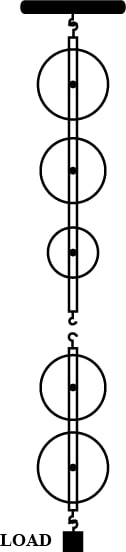(a) Mark in the diagram the direction of load L and effort E.

(b) If the load is raised by 1 m, through what distance will the effort move?

(c) State the number of strands of tackle supporting the load.

(d) What is the mechanical advantage of the system?

(a) The below diagram shows the tackle along with the direction of load L and effort E marked: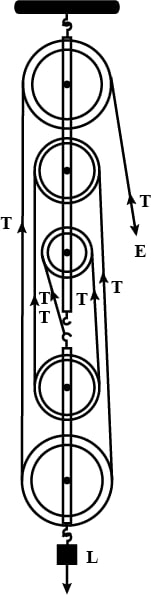(b) As we know that in a system of 5 pulleys, if the load moves up through a distance 1 m, each segment of the string is loosened by a length 1m so the effort end moves through a distance 5 times 1m i.e. 5m

(c) Five strands of tackle are supporting the load.

(d) As we know,

$M.A. = \dfrac{\text {Load}}{\text {Effort}} \\[0.5em]$

Effort = 1m

Substituting the values in the formula for M.A. we get,

$M.A. = \dfrac{5}{1} \\[0.5em] \Rightarrow M.A. = 5 \\[0.5em]$

#### Question 7

A pulley system has a velocity ratio 3. Draw a diagram showing the point of application and direction of load (L), effort (E) and tension (T).

It lifts a load of 150 N by an effort of 60 N.

Is the pulley system ideal? Give reason.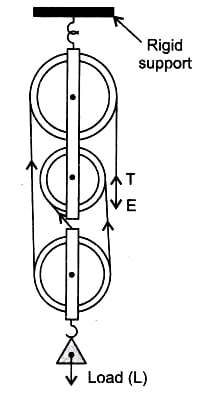Given,

Effort = 60 N

As we know,

$M.A. = \dfrac{\text {Load}}{\text {Effort}} \\[0.5em]$

Substituting the values in the formula for M.A. we get,

$M.A. = \dfrac{150}{60} \\[0.5em] \Rightarrow M.A. = 2.5 \\[0.5em]$

For an ideal case the M.A. should be equal n, where n is the number of pulleys of the pulley system.

In this case as the mechanical advantage is less than 3 ( i.e. the number of pulleys ) so the pulley system is not ideal.

#### Question 8

Figure shows a system of four pulleys. The upper two pulleys are fixed and the lower two are movable.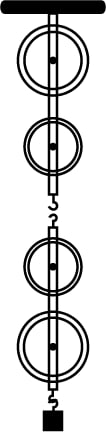(a) Draw a string around the pulleys. Also show the point of application and direction in which the effort E is applied.

(b) What is the velocity ratio of the system?

(c) How are load and effort of the pulley system related?

(d) What assumption do you make in arriving at your answer in part (c)?

(a) The diagram below shows the point of application and direction of the effort E: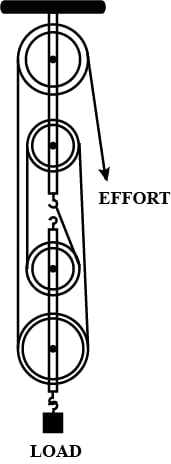(b) As we know that in a system of 4 pulleys, if the load moves up through a distance d, each segment of the string is loosened by a length d so the effort end moves through a distance 4 times d.

$\text{Velocity ratio} = \dfrac{d_E}{d_L} \\[0.5em] \text{Velocity ratio} = \dfrac{4d}{d} \\[0.5em] \Rightarrow \text{Velocity ratio}= 4 \\[0.5em]$

(c) As we know,

$M.A. = \dfrac{\text {Load}}{\text {Effort}} \\[0.5em]$

As M.A. = n = 4

Substituting the values in the formula we get,

$\dfrac{Load}{Effort} = 4 \\[0.5em] \Rightarrow Load = 4 \times Effort$

(i) There is no friction in the pulley bearings.

(ii) Weight of lower pulleys is negligible.

(iii) The effort is applied downwards.

#### Question 9

Figure shows a block and tackle system of pulleys used to lift a load.(a) How many strands of tackle are supporting the load?

(b) Draw arrows to represent tension T in each strand.

(c) What is the mechanical advantage of the system?

(d) When load is pulled up by a distance 1 m, how far does the effort end move?

(e) How much effort is needed to lift a load of 100 N?

(a) There are 4 strands of tackle supporting the load.

(b) Tension T in each strand is as shown in the diagram below: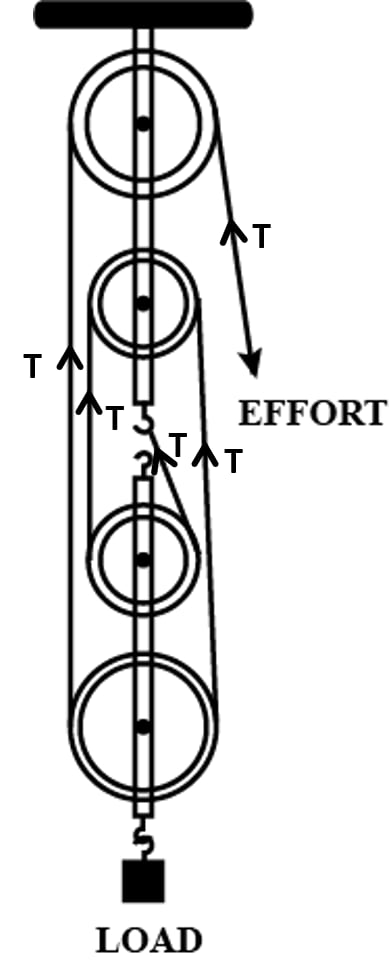(c) As we know,

$M.A. = \dfrac{\text {Load}}{\text {Effort}} \\[0.5em]$

Effort = T

Substituting the values in the formula for M.A. we get,

$M.A. = \dfrac{4T}{T} \\[0.5em]$

Hence, M.A. = 4

(d) In a system of 4 pulleys, if the load moves up through a distance 1m, each segment of the string is loosened by a length 1m so the effort end moves through a distance 4 times 1m. Hence, we get 4m as the distance moved by the effort arm.

(e) As we know,

$M.A. = \dfrac{\text {Load}}{\text {Effort}} \\[0.5em]$

M.A = 4

Substituting the values in the formula for M.A. we get,

$4 = \dfrac{100}{Effort} \\[0.5em] Effort = \dfrac{100}{4} \\[0.5em] \Rightarrow Effort = 25N \\[0.5em]$

#### Question 10

A block and tackle system has the velocity ratio 3. Draw a labelled diagram of the system indicating the points of application and the directions of load L and effort E. A man can exert a pull of 200 kgf.

(a) What is the maximum load he can raise with this pulley system if its efficiency is 60%?

(b) If the effort end moves a distance 60 cm, what distance does the load move?

Given,

V.R = n (number of pulleys) = 3

Hence, number of pulleys = 3

Below is a labelled diagram of the system indicating the points of application and the directions of load L and effort E:(a) As we know,

$\eta = 1 - \dfrac{w}{nE}$

Given,

Effort = 200 kgf

Efficiency = 60% = 0.6

Substituting the values in the formula for efficiency we get,

$0.6 = 1 - \dfrac{w}{3 \times 200} \\[0.5em] \dfrac{w}{600} = 1 - 0.6 \\[0.5em] w = 600 \times 0.4 \\[0.5em] \Rightarrow w = 240 kgf$

As we know,

$Load = (n \times E) - w \\[0.5em] Load = (3 \times 200) - w \\[0.5em] Load = 600 - 240 \\[0.5em] \Rightarrow Load = 360kgf$

(b) The velocity ratio of system is V.R = number of pulleys = 3

As we know,

$V.R. = \dfrac{d_E}{d_L} \\[0.5em]$

Substituting the values in the formula for V.R. we get,

$3 = \dfrac{d_E}{d_L} \\[0.5em] d_L= \dfrac{d_E}{3} \\[0.5em] d_L= \dfrac{60}{3} \\[0.5em] \Rightarrow d_L= 20 cm \\[0.5em]$

#### Question 11

You are given four pulleys and three strings. Draw a neat and labelled diagram to use them so as to obtain a maximum mechanical advantage equal to 8. In your diagram mark the directions of load L, effort E and tension in each strand. What assumptions have you made to obtain the required mechanical advantage?